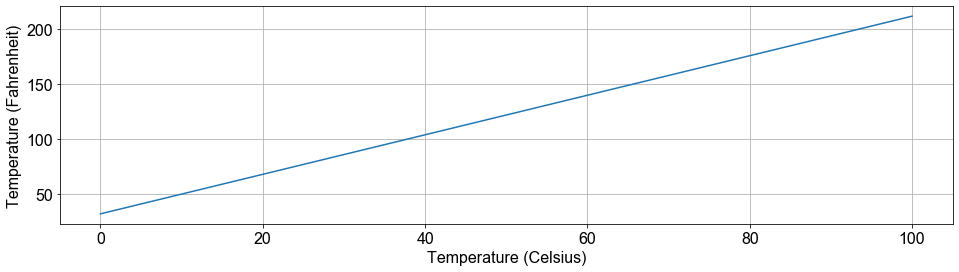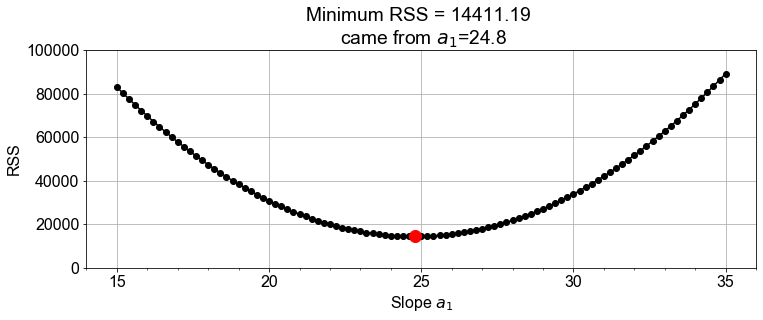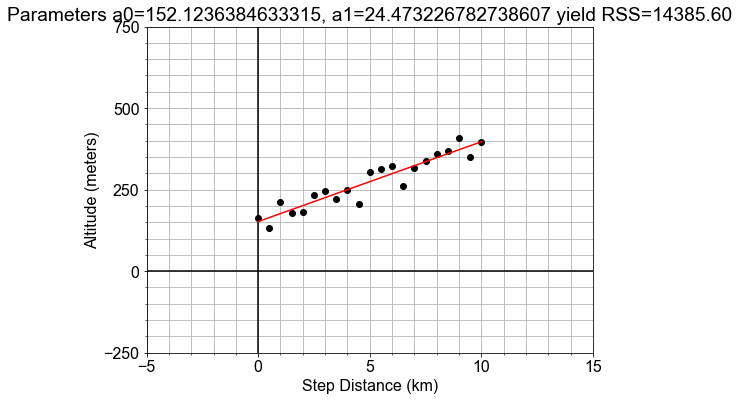import pandas as pd
import numpy as np
import matplotlib.pyplot as plt
import seaborn as sns
import statsmodels.api as sm

plt.rcParams['figure.figsize'] = (10, 5)


## What makes a model linear

• Taylor Series
• Things to know:
1. approximate any curve
2. polynomial form: $y = a_0 + a_1 x + a_2 x^2 + a_3 x^3 + \dots + a_n x^n$
3. often, first order is enough: $y = a_0 + a_1 x$

### Model Components

Previously, you have been given a pre-defined model to work with. In this exercise, you will implement a model function that returns model values for y, computed from input x data, and any input coefficients for the "zero-th" order term a0, the "first-order" term a1, and a quadratic term a2 of a model (see below).

$$y = a_0 + a_1 x + a_2 x_2$$ Recall that "first order" is linear, so we'll set the defaults for this general linear model with a2=0, but later, we will change this for comparison.

def plot_prediction(x, y):
"""
Purpose:
Create a plot of y versus x
Args:
x (np.array): array of values for the indepent variable, e.g. times
y (np.array): array of values for the depedent variable, e.g. distances
Returns:
fig (matplotlib.figure): matplotlib figure object
"""
from matplotlib.ticker import MultipleLocator
font_options = {'family': 'Arial', 'size': 16}
plt.rc('font', **font_options)
fig, axis = plt.subplots()
axis.plot(x, y, color="red", linestyle="-", marker="o")
axis.grid(True, which="both")
axis.axhline(0, color="black")
axis.axvline(0, color="black")
axis.xaxis.set_major_locator(MultipleLocator(5.0))
axis.xaxis.set_minor_locator(MultipleLocator(1.0))
axis.yaxis.set_major_locator(MultipleLocator(5.0))
axis.yaxis.set_minor_locator(MultipleLocator(1.0))
axis.set_ylabel('Y')
axis.set_xlabel('X')
axis.set_title("Plot of modeled Y for given X")
plt.show()
return fig

def model(x, a0=3, a1=2, a2=0):
return a0 + (a1 * x) + (a2 * x * x)

# Generate array x, then predict y values for specific, non-default a0 and a1
x = np.linspace(-10, 10, 21)
y = model(x)

# Plot the results, y versus x
fig = plot_prediction(x, y)### Model Parameters

Now that you've built a general model, let's "optimize" or "fit" it to a new (preloaded) measured data set, xd, yd, by finding the specific values for model parameters a0, a1 for which the model data and the measured data line up on a plot.

This is an iterative visualization strategy, where we start with a guess for model parameters, pass them into the model(), over-plot the resulting modeled data on the measured data, and visually check that the line passes through the points. If it doesn't, we change the model parameters and try again.

def plot_data(x, y):
"""
Purpose:
Create a plot of y versus x
Args:
x (np.array): array of values for the indepent variable, e.g. times
y (np.array): array of values for the depedent variable, e.g. distances
Returns:
fig (matplotlib.figure): matplotlib figure object
"""
from matplotlib.ticker import MultipleLocator
font_options = {'family': 'Arial', 'size': 16}
plt.rc('font', **font_options)
fig, axis = plt.subplots(figsize=(8,6))
axis.plot(x, y, color="black", linestyle=" ", marker="o")
axis.grid(True, which="both")
axis.axhline(0, color="black")
axis.axvline(0, color="black")
axis.set_ylim([-5*50, 15*50])
axis.set_xlim([-5, 15])
axis.xaxis.set_major_locator(MultipleLocator(5.0))
axis.xaxis.set_minor_locator(MultipleLocator(1.0))
axis.yaxis.set_major_locator(MultipleLocator(5.0*50))
axis.yaxis.set_minor_locator(MultipleLocator(1.0*50))
axis.set_ylabel('Altitude (meters)')
axis.set_xlabel('Step Distance (km)')
axis.set_title("Hiking  Trip")
return fig

xd = np.array([  0. ,   0.5,   1. ,   1.5,   2. ,   2.5,   3. ,   3.5,   4. ,
4.5,   5. ,   5.5,   6. ,   6.5,   7. ,   7.5,   8. ,   8.5,
9. ,   9.5,  10. ])

yd = np.array([ 161.78587909,  132.72560763,  210.81767421,  179.6837026 ,
181.98528167,  234.67907351,  246.48971034,  221.58691239,
250.3924093 ,  206.43287615,  303.75089312,  312.29865056,
323.8331032 ,  261.9686295 ,  316.64806585,  337.55295912,
360.13633529,  369.72729852,  408.0289548 ,  348.82736117,
394.93384188])

def plot_data_with_model(xd, yd, ym):
fig = plot_data(xd, yd)  # plot measured data
fig.axes.plot(xd, ym, color='red')  # over-plot modeled data
plt.show()
return fig

# Select new model parameters a0, a1, and generate modeled ym from them.
a0 = 150
a1 = 25
ym = model(xd, a0, a1)

# Plot the resulting model to see whether it fits the data
fig = plot_data_with_model(xd, yd, ym)## Interpreting Slope and Intercept

• Review: Terminology $$y = a_0 + a_1 x$$
• $x$ : independent variable, e.g. time
• $y$ : dependent variable, e.g. distance traveled
• $a_0$ : intercept
• $a_1$ : slope

### Linear Proportionality

The definition of temperature scales is related to the linear expansion of certain liquids, such as mercury and alcohol. Originally, these scales were literally rulers for measuring length of fluid in the narrow marked or "graduated" tube as a proxy for temperature. The alcohol starts in a bulb, and then expands linearly into the tube, in response to increasing temperature of the bulb or whatever surrounds it.

In this exercise, we will explore the conversion between the Fahrenheit and Celsius temperature scales as a demonstration of interpreting slope and intercept of a linear relationship within a physical context.

def plot_temperatures(temps_C, temps_F):
font_options = {'family' : 'Arial', 'size'   : 16}
plt.rc('font', **font_options)
fig, axis = plt.subplots(figsize=(16,4))
axis.plot(temps_C, temps_F)
axis.set_xlabel("Temperature (Celsius)")
axis.set_ylabel("Temperature (Fahrenheit)")
axis.grid()
plt.show()
return fig

def convert_scale(temps_C):
(freeze_C, boil_C) = (0, 100)
(freeze_F, boil_F) = (32, 212)
change_in_C = boil_C - freeze_C
change_in_F = boil_F - freeze_F
slope = change_in_F / change_in_C
intercept = freeze_F - freeze_C
temps_F = intercept + (slope * temps_C)
return temps_F

# Use the convert function to compute values of F and plot them
temps_C = np.linspace(0, 100, 101)
temps_F = convert_scale(temps_C)
fig = plot_temperatures(temps_C, temps_F)### Slope and Rates-of-Change

In this exercise, you will model the motion of a car driving (roughly) constant velocity by computing the average velocity over the entire trip. The linear relationship modeled is between the time elapsed and the distance traveled.

In this case, the model parameter a1, or slope, is approximated or "estimated", as the mean velocity, or put another way, the "rate-of-change" of the distance ("rise") divided by the time ("run").

times = np.array([ 0.        ,  0.08333333,  0.16666667,  0.25      ,  0.33333333,
0.41666667,  0.5       ,  0.58333333,  0.66666667,  0.75      ,
0.83333333,  0.91666667,  1.        ,  1.08333333,  1.16666667,
1.25      ,  1.33333333,  1.41666667,  1.5       ,  1.58333333,
1.66666667,  1.75      ,  1.83333333,  1.91666667,  2.        ])

distances = np.array([   0.13536211,    4.11568697,    8.28931902,   12.41058595,
16.73878397,   20.64153844,   25.14540098,   29.10323276,
33.35991992,   37.47921914,   41.78850899,   45.66165494,
49.9731319 ,   54.13466214,   58.42781412,   62.40834239,
66.65229765,   70.76017847,   75.00351781,   79.2152346 ,
83.24161507,   87.59539364,   91.74179923,   95.87520786,
100.07507133])

def plot_velocity_timeseries(times, velocities):
fig, axis = plt.subplots()
axis.plot(times, velocities, linestyle=" ", marker=".", color='black', label='Velocities')
axis.axhline(np.mean(velocities), color='red', alpha=0.5, lw=4, label='Mean Velocity')
axis.grid(True, which="both")
axis.set_ylabel("Instantaneous Velocity (Kilometers / Hours)")
axis.set_xlabel("Time (Hours)")
axis.set_ylim([0, 100])
fig.tight_layout()
fig.legend(loc='upper center')
plt.show()
return fig

diff_distances = np.diff(distances)
diff_times = np.diff(times)
velocities = diff_distances / diff_times

# Characterize the center and spread of the velocities
v_avg = np.mean(velocities)
v_max = np.max(velocities)
v_min = np.min(velocities)
v_range = v_max - v_min

# Plot the distribution of velocities
fig = plot_velocity_timeseries(times[1:], velocities)### Intercept and Starting Points

In this exercise, you will see the intercept and slope parameters in the context of modeling measurements taken of the volume of a solution contained in a large glass jug. The solution is composed of water, grains, sugars, and yeast. The total mass of both the solution and the glass container was also recorded, but the empty container mass was not noted.

Your job is to use the preloaded pandas DataFrame df, with data columns volumes and masses, to build a linear model that relates the masses (y-data) to the volumes (x-data). The slope will be an estimate of the density (change in mass / change in volume) of the solution, and the intercept will be an estimate of the empty container weight (mass when volume=0).

df = pd.read_csv('./dataset/linear_data.csv', index_col=0)

from statsmodels.formula.api import ols

# Fit a model to the data
model_fit = ols(formula="masses ~ volumes", data=df)
model_fit = model_fit.fit()

# Extract the model parameter values, and assign them to a0, a1
a0 = model_fit.params['Intercept']
a1 = model_fit.params['volumes']

# Print model parameter values with meaningful names, and compare to summary()
print( "container_mass   = {:0.4f}".format(a0) )
print( "solution_density = {:0.4f}".format(a1) )
print( model_fit.summary() )

container_mass   = 5.4349
solution_density = 1.1029
OLS Regression Results
==============================================================================
Dep. Variable:                 masses   R-squared:                       0.999
Method:                 Least Squares   F-statistic:                 1.328e+05
Date:                Fri, 19 Jun 2020   Prob (F-statistic):          1.19e-156
Time:                        17:29:13   Log-Likelihood:                 102.39
No. Observations:                 101   AIC:                            -200.8
Df Residuals:                      99   BIC:                            -195.5
Df Model:                           1
Covariance Type:            nonrobust
==============================================================================
coef    std err          t      P>|t|      [0.025      0.975]
------------------------------------------------------------------------------
Intercept      5.4349      0.023    236.805      0.000       5.389       5.480
volumes        1.1029      0.003    364.408      0.000       1.097       1.109
==============================================================================
Omnibus:                        0.319   Durbin-Watson:                   2.072
Prob(Omnibus):                  0.852   Jarque-Bera (JB):                0.169
Skew:                           0.100   Prob(JB):                        0.919
Kurtosis:                       3.019   Cond. No.                         20.0
==============================================================================

Warnings:
 Standard Errors assume that the covariance matrix of the errors is correctly specified.


## Model Optimization

### Residual Sum of the Squares

In a previous exercise, we saw that the altitude along a hiking trail was roughly fit by a linear model, and we introduced the concept of differences between the model and the data as a measure of model goodness.

In this exercise, you'll work with the same measured data, and quantifying how well a model fits it by computing the sum of the square of the "differences", also called "residuals".

def load_data():
num_pts=21;a0=3.0*50;a1=0.5*50;mu=0;sigma=1;ae=0.5*50;seed=1234;
np.random.seed(seed)
xmin = 0
xmax = 10
x1 = np.linspace(xmin, xmax, num_pts)
e1 = np.array([np.random.normal(mu, sigma) for n in range(num_pts)])
y1 = a0 + (a1*x1) + ae*e1
return x1, y1

def model(x, a0=150, a1=25):
"""
Purpose:
For a given measured data x, compute the model prediction for y
The form of the model is y = a0 + a1*x
Args:
x (float, np.ndarray): independent variable, e.g. time
a0 (float): default=150, coefficient for the Zeroth order term in the model, i.e. a0
a1 (float): default=25, coefficient for the 1st order term in the model, i.e. a1*x
Returns:
y (float, np.ndarray): model values predicted for corresponding input x.
"""
y = a0 + (a1*x)
return y

x_data, y_data = load_data()

# Model the data with specified values for parameters a0, a1
y_model = model(x_data, a0=150, a1=25)

# Compute the RSS value for this parameterization of the model

RSS = 14444.484117694472


### Minimizing the Residuals

In this exercise, you will complete a function to visually compare model and data, and compute and print the RSS. You will call it more than once to see how RSS changes when you change values for a0 and a1. We'll see that the values for the parameters we found earlier are the ones needed to minimize the RSS.

def plot_data_with_model_and_title(xd, yd, ym, title):
fig = plot_data(xd, yd)
fig.axes.plot(xd, ym, color="red")
fig.axes.set_title(title)
plt.show()
return fig

def compute_rss_and_plot_fit(a0, a1):
ym = model(xd, a0, a1)
residuals = ym - yd
fig = plot_data_with_model_and_title(xd, yd, ym, summary)

# Chose model parameter values and pass them into RSS function
print(summary)Parameters a0=150, a1=25 yield RSS=14444.48


In this exercise you will compute and visualize how RSS varies for different values of model parameters. Start by holding the intercept constant, but vary the slope: and for each slope value, you'll compute the model values, and the resulting RSS. Once you have an array of RSS values, you will determine minimal RSS value, in code, and from that minimum, determine the slope that resulted in that minimal RSS.

def compute_rss(yd, ym):

def plot_rss_vs_a1(a1_array, rss_array):
"""
Purpose:
Plot RSS values (y-axis) versus a1 parameters values (x-axis)
Also plot a point where the minimum RSS value occurs, and the
corresponding a1 value whose model resulted in that minimum RSS.
Args:
a1_array (np.array): an array of trial values for a1 (model slope)
rss_array (np.array): an array of computed RSS values resulting from the a1_array
Returns:
fig (matplotlib.figure): figure object on which the data is plotted
"""
from matplotlib.ticker import MultipleLocator
font_options = {'family': 'Arial', 'size': 16}
plt.rc('font', **font_options)
fig, axis = plt.subplots(figsize=(12,4))
axis.plot(best_slope, min_rss, marker="o", markersize=12, linestyle=" ", color='red')
axis.xaxis.set_major_locator(MultipleLocator(5.0))
axis.xaxis.set_minor_locator(MultipleLocator(1.0))
axis.grid(True, which="major")
axis.set_xlabel("Slope $a_1$")
axis.set_ylim([0,100000])
axis.set_title("Minimum RSS = {:.02f} \n came from $a_1$={}".format(min_rss, best_slope))
plt.show()
return fig

rss_list = []

a1_array = np.linspace(15, 35, 101)
for a1_trial in a1_array:
y_model = model(x_data, a0=150, a1=a1_trial)

# Find the minimum RSS and the a1 value from whence it came


The minimum RSS = 14411.193019771845, came from a1 = [24.8]## Least-Squares Optimization

### Least-Squares with numpy

The formulae below are the result of working through the calculus discussed in the introduction. In this exercise, we'll trust that the calculus correct, and implement these formulae in code using numpy.

$$a_1 = \frac{covariance(x, y)}{variance(x)}$$ $$a_0 = mean(y) - a_1 mean(x)$$

x_mean = np.mean(x_data)
y_mean = np.mean(y_data)
x_dev = x_data - x_mean
y_dev = y_data - y_mean

# Complete least-squares formulae to find the optimal a0, a1
a1 = np.sum(x_dev * y_dev) / np.sum( np.square(x_dev) )
a0 = y_mean - (a1 * x_mean)

# Use the those optimal model parameters a0, a1 to build a model
y_model = model(x_data, a0, a1)

# plot to verify that the resulting y_model best fits the data y### Optimization with Scipy

It is possible to write a numpy implementation of the analytic solution to find the minimal RSS value. But for more complex models, finding analytic formulae is not possible, and so we turn to other methods.

In this exercise you will use scipy.optimize to employ a more general approach to solve the same optimization problem.

In so doing, you will see additional return values from the method that tell answer us "how good is best". Here we will use the same measured data and parameters as seen in the last exercise for ease of comparison of the new scipy approach.

from scipy import optimize

# Define a model function needed as input to scipy
def model_func(x, a0, a1):
return a0 + (a1 * x)

# Load the measured data you want to model

# call curve_fit, passing in the model function and data; then unpack the results
param_opt, param_cov = optimize.curve_fit(model_func, x_data, y_data)
a0 = param_opt # a0 is the intercept in y = a0 + a1*x
a1 = param_opt # a1 is the slope     in y = a0 + a1*x

# test that these parameters result in a model that fits the data### Least-Squares with statsmodels

Several python libraries provide convenient abstracted interfaces so that you need not always be so explicit in handling the machinery of optimization of the model.

As an example, in this exercise, you will use the statsmodels library in a more high-level, generalized work-flow for building a model using least-squares optimization (minimization of RSS).

from statsmodels.formula.api import ols

df = pd.DataFrame(dict(x_column=x_data, y_column=y_data))

# Pass data and 'formula' into ols(), use and '.fit()' the model to the data
model_fit = ols(formula='y_column ~ x_column', data=df).fit()

# Use .predict(df) to get y_model values, then over-plot y_data with y_model
y_model = model_fit.predict(df)
fig = plot_data_with_model(x_data, y_data, y_model)

# Extract the a0, a1 values from model_fit.params
a0 = model_fit.params['Intercept']
a1 = model_fit.params['x_column']

# Visually verify that these parameters a0, a1 give the minimum RSS